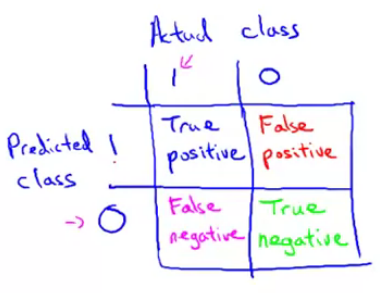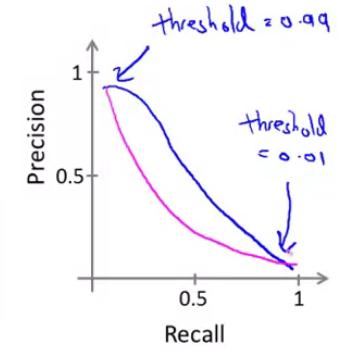# How to debug your machine learning system

Last updated on：a month ago

Sometimes it’s difficult for a newcomer to build their first machine learning system. So, I want to write down some notes from my ML classes to give me some cues to construct the system.

# General advice for debugging a learning algorithm

• Get more training examples (not always works)
• Try smaller sets of features
• Try decreasing/increasing lambda

• Collect lots of data
• Develop sophisticated features based on email routing information (from email header)
• Develop sophisticated features for the message body, features about punctuation
• Develop a sophisticated algorithm to detect misspelling

It is difficult to tell which of the options will be most helpful.

## Error analysis

Recommended approach

• Start with a simple algorithm that you can implement quickly implement and test it on your cross-validation data

• Plot learning curves to decide if more data, more features, etc. are likely to help

• Error analysis: Manually examine the examples (in cross validation set) that your algorithm made errors on. See if you spot any systematic trend in what type of examples it is making errors on.

Error analysis may help decide if this is likely to improve performance. The only solution is to try it and see if it works.

## Error matrices for skewed classes

• Accuracy = (true positives + true negatives) / (total examples)

• Precision = (true positives) / (true positives + false positives)

• Recall = (true positives) / (true positives + false negatives)

• F1 score (F score) = $2\frac{PR}{P+R}$ or $\frac{2}{1/P+1/R}$Using a single number evaluation metric. dev set + single real number of evaluation matrix.

Algorithm US China India Other Average
A 3% 7% 5% 9% 6%
B 5% 6% 5% 10% 6.5%
C 2% 3% 4% 5% 3.5%
D 5% 8% 7% 2% 5.25%
E 4% 5% 2% 4% 3.75%
F 7% 11% 8% 12% 9.5%

## Satisficing and optimizing metrics

eg. maximize accuracy + running time <= 100ms
N metrics: 1 optimizing, N-1 satisficing
Wake-words/trigger words

Classifier Accuracy Running time
A 90% 80ms
B 92% 95ms
C 95% 1500ms

When to change dev/test sets and metrics

Metric: classification error
algorithm A: 3% error -> pornographic (Metric + dev)
algorithm B 5% error (you/users choose)
error: $$\frac{1}{m_{dev}} \sum^{m_{dev}}{i=1} w^{(i)} L{y^{(i)}{predict} \neq y^{(i)}}$$

$$\omega^{(i)} = \begin{cases} 1; \text{if} \ x^{(i)} \text{is non-porn} \\ 10; \text{if} \ x^{(i)} \text{is porn} \end{cases}$$

Step

• Place the target
• Aim/shot at the target

If doing well on your metric + dev /test set does not correspond to doing well on your application, change your metric and/or dev/test set.

## Data for machine learning

• Use a learning algorithm with many parameters; neural network with many hidden layers — low bias
• Use a relatively large training set – low variance
• Choose a dev set and test set to reflect data you expect to get in the future and consider important to do well on.

Set your test set to be big enough to give high confidence in the overall performance of your system. Maybe without test set.

# Reference

 Andrew NG, Machine learning